# Divisors

The sum of all divisors unknown odd number is 2112. Determine sum of all divisors of number which is twice of unknown numbers.

Correct result:

x =  6336

#### Solution: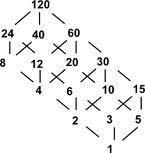We would be pleased if you find an error in the word problem, spelling mistakes, or inaccuracies and send it to us. Thank you!## Next similar math problems:

• One halfOne half of ? is: ?
• PowersExpress the expression ? as the n-th power of the base 10.
• Seamstress 2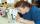Seamstress bought two kinds of textile in whole meters. One at 50 SKK and the second 70 SKK per meter. How many meter bought from this two textiles when paid totally 1540 SKK?
• Nineteenth memberFind the nineteenth member of the arithmetic sequence: a1=33 d=5 find a19
• 6 termsFind the first six terms of the sequence. a1 = 7, an = an-1 + 6
• The diceWhat is the probability of events that if we throw a dice is rolled less than 6?
• AntennasIf you give me two antennas will be same. If you give me again your two antenna I have a 5× so many than you. How many antennas have both mans?
• Toy carsPavel has a collection of toy cars. He wanted to regroup them. But in the division of three, four, six, and eight, he was always one left. Only when he formed groups of seven, he divided everyone. How many toy cars have in the collection?
• Three friendsThe three friends spent 600 KC in a teahouse. Thomas paid twice as much as Paul. Paul a half less than Zdeněk. How many each paid?
• CandiesIf Alena give Lenka 3 candy will still have 1 more candy. If Lenka give Alena 1 candy Alena will hame twice more than Lenka. How many candies have each of them?
• Numbers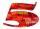Write smallest three-digit number, which in division 5 and 7 gives the rest 2.
• CombinationsHow many different combinations of two-digit number divisible by 4 arises from the digits 3, 5 and 7?
• Repair company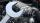The company repairs cars. The first day repair half of the contract second day, the half of the rest and third day 8 residue cars. How many total cars company repaired?In six baskets, the seller has fruit. In individual baskets, there are only apples or just pears with the following number of fruits: 5,6,12,14,23 and 29. "If I sell this basket," the salesman thinks, "then I will have just as many apples as a pear." Whic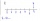Find four-digit numbers where all the digits are different. For numbers, the sum of the third and fourth digits is twice the sum of the first two digits, and the sum of the first and fourth digits is equal to the sum of the second and third digits. The diAn amazing number is name for such even number, the decomposition product of prime numbers has exactly three not necessarily different factors and the sum of all its divisors is equal to twice that number. Find all amazing numbers.Unknown number is divisible by exactly three different primes. When we compare these primes in ascending order, the following applies: • Difference first and second prime number is half the difference between the third and second prime numbers. • The prod